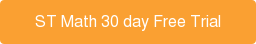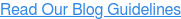### Subscribe to Our BlogFeatured Podcast

Listen Now

# 4 Steps to Student Success With Word Problems Using ST Math

“Word problems are easy to teach!” said no teacher ever.

We all know the truth - word problems can be wonderful opportunities for students to apply deep mathematical knowledge toward complex real world problems.

But getting students there is the hard part.

When it comes to tackling the challenge of teaching word problems, research can help guide us in the right direction. Here are four small but significant changes we can make to support students through word problems.

## Step 1: Focus on Conceptual, Foundational Knowledge

Conceptual understanding opens the door to higher order thinking and complex problem solving. In order to be successful with word problems, students must have a concrete understanding of abstract mathematical concepts.

ST Math’s interactive visual approach builds conceptual understanding of foundation concepts so that students develop lasting knowledge. Here are three foundational math concepts and activity examples from ST Math.

 Concept Leads to... ST Math Puzzle Fair Sharing Division & MultiplicationStudents must move boats to make an equal number on both sides of the bridge. Rates & RatiosStudents must determine how many monsters will eat 10 pears if each one eats 2. Base Ten Number SenseUsing the ten frame, students must add 8 and 1. OperationsStudents must determine how many tens & ones are needed to add to 42 in order to make 100. Patterns Algebraic FunctionsGiven the number pattern, students must determine the missing value.

## Step 2: Teach Students to Visualize

Research shows that giving students opportunity to practice visualizing math has significant effect on achievement. By teaching students this crucial strategy, they learn to better understand what the problem is asking.

“When students learn through visual approaches, mathematics changes for them, and they are given access to deep and new understandings.” -Jo Boaler, Seeing as Understanding: The Importance of Visual Mathematics for our Brain and Learning

Think of how successful a student would be with this sample 3rd grade high-stakes test question if they were able to visualize the scenario.

Sample Test Question: Cade has four boxes. He puts 9 model cars in each box. What is the total number of model cars he put in the boxes?Teaching students to visualize complex problems will increase achievement.

The best way to teach students to visualize math concepts is to teach math concepts visually.  Educators can utilize a number of resources to help students visualize math. Here’s how ST Math uses a picture to teach a similar concept to the sample assessment question above:This puzzle asks the question, if 5 snails share 15 blocks,
how many will each snail get? Here the student has selected 3.

Sources: Seeing as Understanding: The Importance of Visual Mathematics for our Brain and Learning & Partnership for Assessment of Readiness for College and Careers

## Step 3: Develop Strategies Instead of Procedures

It’s easy to fall into the practice of proceduralizing word problems. But teaching keywords or step-by-step processes contradicts the very reason we give students word problems in the first place - to promote real-world application and higher order thinking.

Instead of asking students to memorize word problem procedures, we should equip them with applicable strategies. Here are four problem solving strategies that can help students find solutions to rigorous word problems.Look for patterns Estimate, check and improve Solve a simpler problem Break problem into smaller parts

## Step 4: Explore Multiple Pathways to Solutions

Most word problems offer many different pathways to the solution. It is important that we teach students early on that they are free to apply their own unique thought process when faced with problems.

By providing opportunities for students to share their reasoning and celebrating their different ways thinking, teachers will encourage creativity and confidence to take on any challenge.

Consider how this 5th grade sample high-stakes test question could be solved in multiple different ways.

Sample Test Question: The rectangular prism shown has 4 layers with 6 cubes in each layer. What is the volume, in cubic centimeters, of the rectangular prism?To solve the problem, students must have a conceptual understanding of the question and then employ their own creative solution. The graphic above shows three ways to visualize the solution to the problem.

ST Math contains interactive visual puzzles that empower students to take their own pathways to find solutions, similar to the sample assessment question above.In this puzzle, students need to select the volume within the cylinder that matches the volume of the box. Here the student has selected 24.

While there may not be a silver bullet when it comes to word problems, with a few small changes, we can make a big difference!

Learn how ST Math’s research and studies continue to reveal the program’s powerful impact on math education and try it for your students!